# RNN

RNN（Recurrent Neural Networks,循环神经网络）不仅会学习当前时刻的信息，也会依赖之前的序列信息。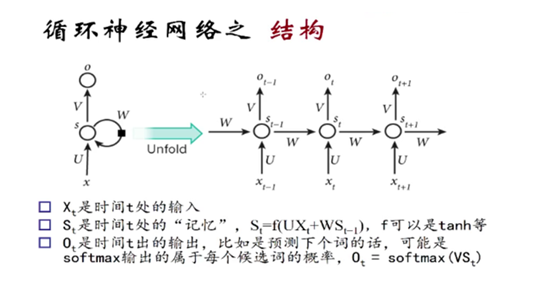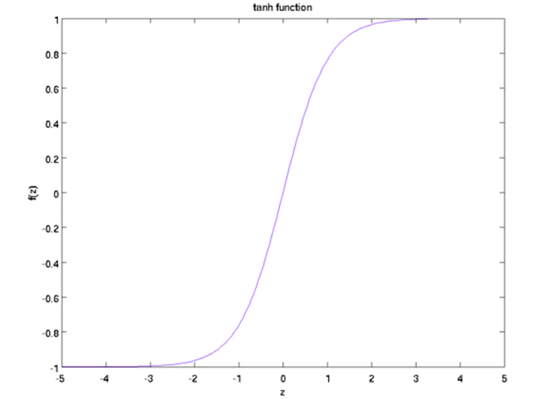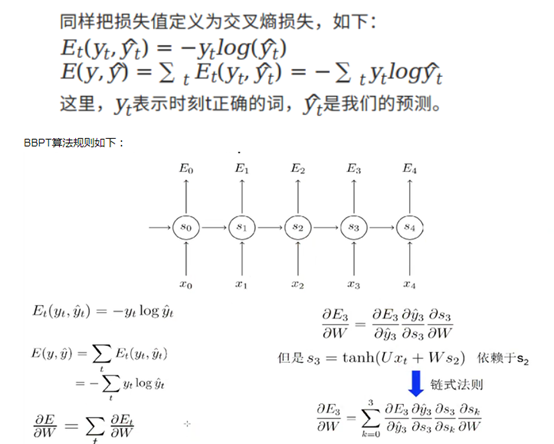RNN的激活函数tanh可以将所有值映射到-1至1之间，以及在利用梯度下降算法调优时利用链式法则，那么会造成很多个小于1的项连乘就很快的逼近零。

# LSTM

LSTM (Long Short Term Memory networks)的“门”结构可以截取“不该截取的信息”，结构如下：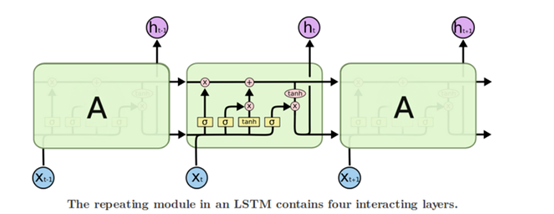LSTMs核心理念

LSTMs的关键点是细胞状态，就是穿过图中的水平线。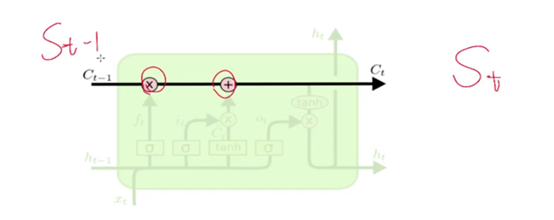LSTM有能力向单元状态中移除或添加信息，通过门结构来管理，包括“遗忘门”，“输出门”，“输入门”。通过门让信息选择性通过，来去除或增加信息到细胞状态. 模块中sigmoid层输出0到1之间的数字，描述了每个成分应该通过门限的程度。0表示“不让任何成分通过”，而1表示“让所有成分通过！”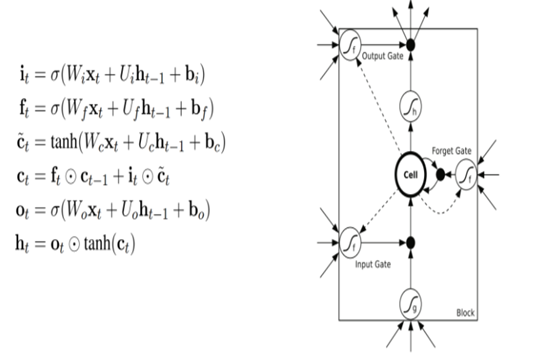LSTM循环神经网络分步说明: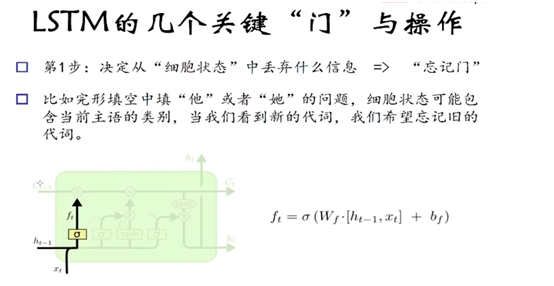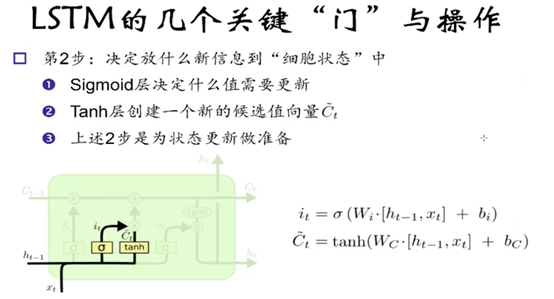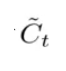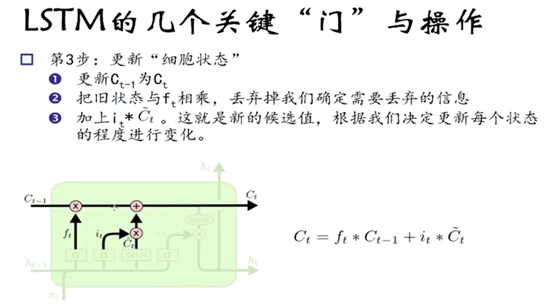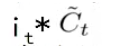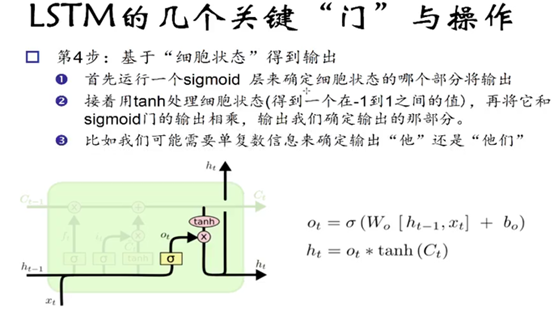Sigmoid函数的输出是不考虑先前时刻学到的信息的输出，tanh函数是对先前学到的信息的压缩处理，起到稳定数值的作用，两者的结合就是循环神经网络的学习思想。

# 代码演示

``````"""
demo 使用单层 LSTM网络 对 MNIST数据集分类

RNN网络应用领域：

lstm 长短期记忆网络
gru 闸门重复单元
"""

import tensorflow as tf
import numpy as np
# 导入 MINST 数据集
from tensorflow.examples.tutorials.mnist import input_data
mnist = input_data.read_data_sets("MNIST_data/", one_hot=True) # 在线下载 MNIST_data 数据集

n_input = 28 # MNIST data 输入 (img shape: 28*28)
n_steps = 28 # 序列个数
n_hidden = 128 # hidden layer num of features
n_classes = 10  # MNIST 列别 (0-9 ，一共10类)

tf.reset_default_graph()

# tf Graph input
x = tf.placeholder("float", [None, n_steps, n_input])
y = tf.placeholder("float", [None, n_classes])

x1 = tf.unstack(x, n_steps, 1)

#1 BasicLSTMCell  0.9453125
lstm_cell = tf.contrib.rnn.BasicLSTMCell(n_hidden, forget_bias=1.0)
outputs, states = tf.contrib.rnn.static_rnn(lstm_cell, x1, dtype=tf.float32)

#2 LSTMCell  0.9609375
# lstm_cell = tf.contrib.rnn.LSTMCell(n_hidden, forget_bias=1.0)
# outputs, states = tf.contrib.rnn.static_rnn(lstm_cell, x1, dtype=tf.float32)

#3 gru 0.9921875
# gru = tf.contrib.rnn.GRUCell(n_hidden)
# outputs = tf.contrib.rnn.static_rnn(gru, x1, dtype=tf.float32)

#4 创建动态RNN 0.9921875
# outputs,_  = tf.nn.dynamic_rnn(gru,x,dtype=tf.float32)
# outputs = tf.transpose(outputs, [1, 0, 2])

pred = tf.contrib.layers.fully_connected(outputs[-1],n_classes,activation_fn = None)

learning_rate = 0.001
training_iters = 100000
batch_size = 128
display_step = 10

# Define loss and optimizer
cost = tf.reduce_mean(tf.nn.softmax_cross_entropy_with_logits(logits=pred, labels=y))

# Evaluate model
correct_pred = tf.equal(tf.argmax(pred,1), tf.argmax(y,1))
accuracy = tf.reduce_mean(tf.cast(correct_pred, tf.float32))

# 启动session
with tf.Session() as sess:
sess.run(tf.global_variables_initializer())
step = 1
# Keep training until reach max iterations
while step * batch_size < training_iters:
batch_x, batch_y = mnist.train.next_batch(batch_size)
# Reshape data to get 28 seq of 28 elements
batch_x = batch_x.reshape((batch_size, n_steps, n_input))
# Run optimization op (backprop)
sess.run(optimizer, feed_dict={x: batch_x, y: batch_y})
if step % display_step == 0:
# 计算批次数据的准确率
acc = sess.run(accuracy, feed_dict={x: batch_x, y: batch_y})
# Calculate batch loss
loss = sess.run(cost, feed_dict={x: batch_x, y: batch_y})
print ("Iter " + str(step*batch_size) + ", Minibatch Loss= " + \
"{:.6f}".format(loss) + ", Training Accuracy= " + \
"{:.5f}".format(acc))
step += 1
print (" Finished!")

# 计算准确率 for 128 mnist test images
test_len = 128
test_data = mnist.test.images[:test_len].reshape((-1, n_steps, n_input))
test_label = mnist.test.labels[:test_len]
print ("Testing Accuracy:", \
sess.run(accuracy, feed_dict={x: test_data, y: test_label}))
``````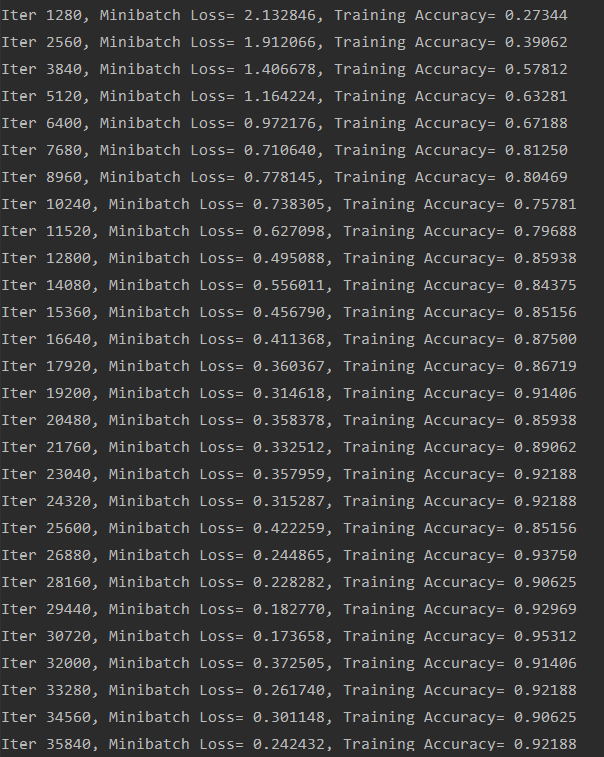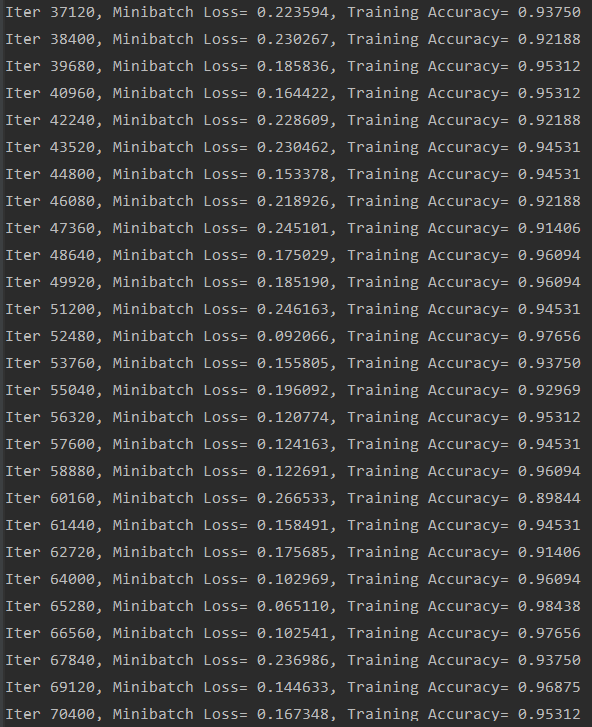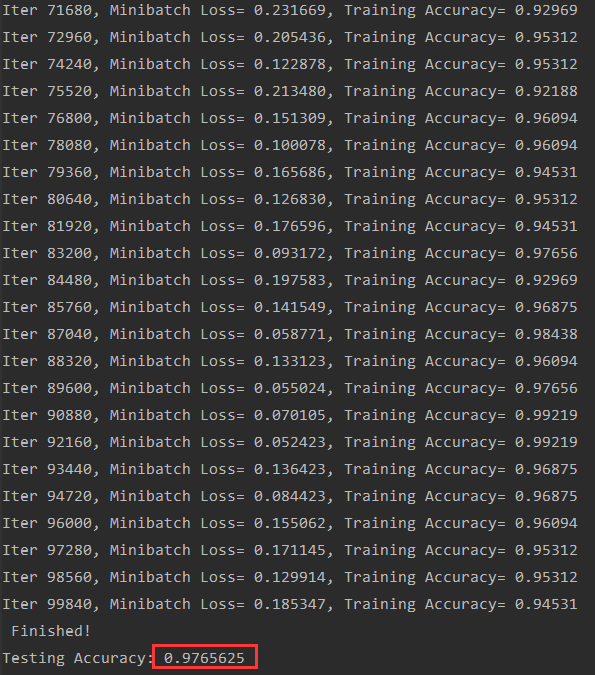### ericSM

Tensorflow 入门教程

Tensorflow 入门教程 http://tensornews.cn/ 深度学习发展史 特征工程 深度学习之激活函数 损失函数 反向传播算法 [上] 反向传播算法 [下] Tensorflow 介绍和安装 Tensorflow 基本操作 卷积神...

07/08
0
0

《Tensorflow基础快速入门》课程的目的是帮助广大的深度学习爱好者，逐层深入，步步精通当下最流行的深度学习框架Tensorflow。该课程包含Tensorflow运行原理，Tensor上面常见的操作，常见API...

liwei2000
2018/07/05
83
0

Image source from unsplash by Timothy L Brock 上一篇文章介绍了如何在 Keras 中调用 Tensorboard。这篇文章就来谈谈如何用 Tensorboard 帮助模型调参。 代码repo见这里 https://github.c...

Hongtao洪滔
06/19
0
0

2018/07/04
0
0
【AI实战】快速掌握TensorFlow（三）：激励函数

2018/08/30
1K
0

import pandas as pdimport matplotlib.pyplot as pltimport matplotlib as mp1mp1.rcParams["font.family"] = "STFangsong"# 加载《销售》表数据df1 = pd.read_excel(r"C:\Us......

5
0
OSChina 周五乱弹 —— 一次四千 要4次还能多给一千

Osc乱弹歌单（2019）请戳（这里） 【今日歌曲】 @这次装个文艺青年吧 ：#今日歌曲推荐# 分享金志文的单曲《远走高飞》: 版权又回来了现在听歌得好几个软件 《远走高飞》- 金志文 手机党少年们...

7
0
Spring Cloud Alibaba 实战(十) - Spring Cloud GateWay

> 本文主要内容是:为什么要使用网关,整合Gateway,Gateway核心学习:Route,Predicate,Filter,最后使用Gateway聚合微服务请求 先总结至此的架构 1 网关的价值 不使用网关行嘛? 各个请求直接打在...

JavaEdge

4
0
【CKB.DEV 茶话会】第二期：聊聊 CKB 钱包和 Nervos DAO 全流程

CKB.DEV 茶话会第二期：聊聊 CKB 钱包和 Nervos DAO 全流程 为了鼓励更多优秀的开发者和研究人员参与到 CKB 的开发和生态建设中去，我们希望组织一系列 CKB Developer Seminar（CKB.DEV 茶话...

NervosCommunity

4
0

go4it

4
0